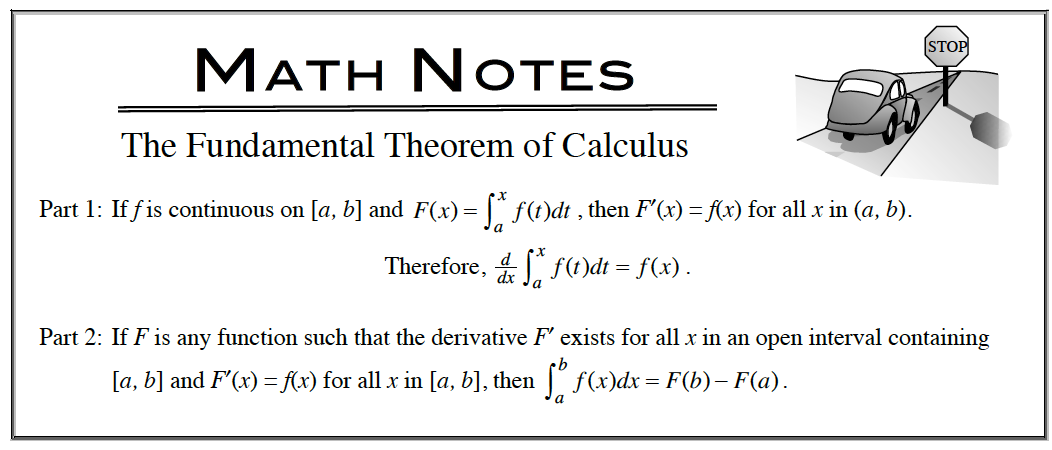### Home > APCALC > Chapter 5 > Lesson 5.2.2 > Problem5-72

5-72.

Evaluate the following integrals without a calculator. Homework Help ✎1. $\int _ { - 1 } ^ { - 1 } ( - 9 t ^ { 6 / 5 } - 15 t ^ { - 11 / 3 } ) d t$

Fundamental Theorem of Calculus, Part 22. $\int _ { 0 } ^ { 2 } \frac { d } { d x } ( 9 x - 1 ) d x$

Fundamental Theorem of Calculus, Part 1

3. $\int ( - 32 t + 12 ) d t$

$−16t^2 + 12t + C$

4. $\int \operatorname { cos } ( 5 x ) d x$

$k \sin(5x) + C$
Determine $k$.

5. $\int _ { 1 } ^ { - 1 } ( 4 x ^ { 2 } - 6 x ) d x + \int _ { 1 } ^ { 2 } ( 2 x ^ { 2 } - 3 x ) d x$

$\frac{4}{3}x^3-3x^2\Big|_1^{-1}-\frac{2}{3}x^3-\frac{3}{2}x^2\Big|_1^2=-\frac{7}{3}$